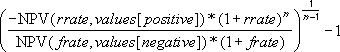# Everyday Calculation

Free calculators and unit converters for general and everyday use.

Calculators » Financial » MIRR

## MIRR Calculator

Our online tools will provide quick answers to your calculation and conversion needs. On this page, you can calculate modified internal rate of return (MIRR) of an investment based on a series of cash flows that occur at regular time intervals. Cash flows may be set to annual, semi-annual, quarterly, monthly, weekly or daily intervals.

% p.a.

% p.a.

Cash flow

× ( )

Result window

Android: Use this MIRR calculator offline with our TVM financial calculator app.

#### MIRR calculation

We use the same formula as implemented in MS-Excel for calculating modified internal rate of return. The formula of MIRR is:where, n is the number of cash flows

Both the finance rate frate and reinvestment rate rrate are periodic rates. In this calculator, you enter rates as annual percentage rate; they will be automatically converted to their equivalent periodic rate depending on the frequency of payment.

For explanation of MIRR function, refer this MS-excel document.